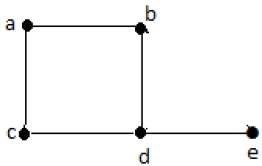# Graph Theory - Introduction

In the domain of mathematics and computer science, graph theory is the study of graphs that concerns with the relationship among edges and vertices. It is a popular subject having its applications in computer science, information technology, biosciences, mathematics, and linguistics to name a few. Without further ado, let us start with defining a graph.

## What is a Graph?

A graph is a pictorial representation of a set of objects where some pairs of objects are connected by links. The interconnected objects are represented by points termed as vertices, and the links that connect the vertices are called edges.

Formally, a graph is a pair of sets (V, E), where V is the set of vertices and E is the set of edges, connecting the pairs of vertices. Take a look at the following graph −In the above graph,

V = {a, b, c, d, e}

E = {ab, ac, bd, cd, de}

## Applications of Graph Theory

Graph theory has its applications in diverse fields of engineering −

Electrical Engineering − The concepts of graph theory is used extensively in designing circuit connections. The types or organization of connections are named as topologies. Some examples for topologies are star, bridge, series, and parallel topologies.

Computer Science − Graph theory is used for the study of algorithms. For example,

• Kruskal's Algorithm
• Prim's Algorithm
• Dijkstra's Algorithm

Computer Network − The relationships among interconnected computers in the network follows the principles of graph theory.

Science − The molecular structure and chemical structure of a substance, the DNA structure of an organism, etc., are represented by graphs.

Linguistics − The parsing tree of a language and grammar of a language uses graphs.

General − Routes between the cities can be represented using graphs. Depicting hierarchical ordered information such as family tree can be used as a special type of graph called tree.